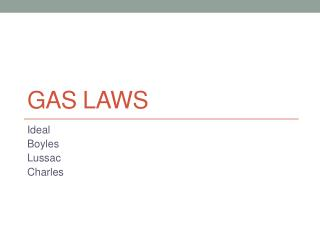DownloadDownload PresentationGas Laws

# Gas Laws

Download Presentation## Gas Laws

- - - - - - - - - - - - - - - - - - - - - - - - - - - E N D - - - - - - - - - - - - - - - - - - - - - - - - - - -
##### Presentation Transcript

1. Gas Laws Ideal Boyles Lussac Charles

2. Variables that describe a Gas P= Pressure T = Temperature (MUST be in Kelvin!) n= Amount (moles) V= Volume (MUST be in Liters!!)

3. Gas Laws

4. Boyles Law=Temp is Constant

5. Lussac’s Law= Volume Constant

6. Charles Law= Pressure Constant

7. Combined Gas Law

8. 1. A 550.0 mL sample of nitrogen gas is warmed from 77.0C to 80.0C. Find the new volume if the pressure remains constant. What law does this situation follow?

9. 2. Convert 338L at 6.38 x 103kPa to its new volume at standard pressure. What law does this situation follow?

10. 3. A gas has a pressure if 0.0370 atm at 50.0C. What is the pressure at standard temperature?

11. Ideal gas law

12. Ideal Gas Law PV =nRT R= gas constant R =8.31 LkPaR=0.0821 Latm moleKmoleK R=62.4 mmHgL all other units can mole Kbe converted

13. 4. Calculate the pressure, in atmospheres, exerted by each of the following: a. 250L of gas containing 1.35 moles at 320K b. 4.75L of gas containing 0.86 moles at 300K.

14. 5. Calculate the volume, in liters, occupied by each of the following: a. 4.0 grams of H2 at 300K and 1.25 atm. b. 0.425 moles of ammonia gas (NH3) at 10.5 PSI and 37C.

15. 6. Determine the amount: a. In moles, of 1.25L of O2 at 805.6 mmHg and 250K. b. In grams, of 0.80L of ammonia gas at 0.925 atm and 27C. answer: 0.51g

16. Ok now your turn to practice: Answers Don’t forget: 1 atm= 760mmHg(torr)=101.3kPa=14.7psi °C= 5/9 (°F-32)